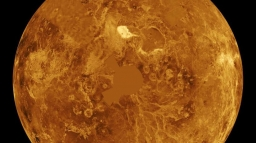On a

On a some day, the Sun, Venus and the Earth are in eclipse, i. E. Venus is between the Sun and the Earth. Venus orbits the Sun in 225 days. In how many years will all three bodies be in alignment again?

x =  1.6071

Step-by-step explanation:Did you find an error or inaccuracy? Feel free to write us. Thank you!Tips to related online calculators
Do you want to calculate least common multiple two or more numbers?
Do you solve Diofant problems and looking for a calculator of Diofant integer equations?
Do you want to convert velocity (speed) units?
Do you want to convert time units like minutes to seconds?

You need to know the following knowledge to solve this word math problem:

We encourage you to watch this tutorial video on this math problem: